Please wait a minute...
 Chin. Phys. B, 2020, Vol. 29(11): 115202    DOI: 10.1088/1674-1056/ab9c14
 PHYSICS OF GASES, PLASMAS, AND ELECTRIC DISCHARGES Prev   Next

# Weakly nonlinear multi-mode Bell–Plesset growth in cylindrical geometry

Hong-Yu Guo(郭宏宇)1,2, †, Tao Cheng(程涛)1,2, and Ying-Jun Li(李英骏)1,2,, ‡
1 State Key Laboratory for Geomechanics and Deep Underground Engineering, China University of Mining and Technology, Beijing 100083, China
2 School of Science, China University of Mining and Technology, Beijing 100083, China
 Abstract  Bell–Plesset (BP) effect caused perturbation growth plays an important role in better understanding of characteristics of the convergence effect. Governing equations for multi-mode perturbation growth on a cylindrically convergent interface are derived. The second-order weakly nonlinear (WN) solutions for two-mode perturbations at the interface which is subject to uniformly radical motion are obtained. Our WN theory is consistent with the numerical result in terms of mode-coupling effect in converging Richtmyer–Meshkov instability. Nonlinear mode-coupling effects will cause irregular deformation of the convergent interface. The mode-coupling behavior in convergent geometry depends on the mode number, Atwood number A and convergence ratio Cr. The A = –1.0 at the interface results in larger perturbation growth than A = 1.0. The growth of generated perturbation modes from two similar modes at the initial stage are smaller than that from two dissimilar modes. Keywords:  Bell-Plesset effect      Rayleigh-Taylor instability      inertial confinement fusion Received:  26 April 2020      Revised:  07 June 2020      Accepted manuscript online:  12 June 2020 Fund: the Fundamental Research Funds for the Central Universities, China (Grant No. 2019QS04), the National Natural Science Foundation of China (Grant No. 11574390), and the Strategic Priority Research Program of Chinese Academy of Sciences (Grant No. XDA 25051000). Corresponding Authors:  †Corresponding author. E-mail: ghy@cumtb.edu.cn ‡Corresponding author. E-mail: lyj@aphy.iphy.ac.cn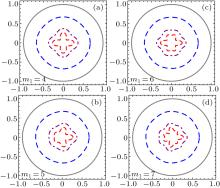Fig. 1.  Evolution of interfacial profiles for two-mode perturbations at Cr = 1 (solid), 1.5 (dashed), 3 (dot-dashed) and 5 (dashed) with A = 1.0. The mode numbers are m1 = 4 (a), 5 (b), 6 (c) and 7 (d), m2 = 4. The initial velocity perturbations are ${\dot{\alpha }}_{{m}_{1}}={\dot{\alpha }}_{{m}_{2}}=0.01{V}_{0}$.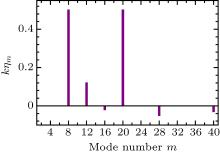Fig. 2.  Normalized perturbation amplitudes for the initial two-mode perturbation at convergent ratio Cr = 3.5. The equivalent wavenumber is k = m/R, the other parameters are A = 1.0, m1 = 20, m2 = 8 and ${\dot{\alpha }}_{{m}_{1}}={\dot{\alpha }}_{{m}_{2}}=0.01{V}_{0}$.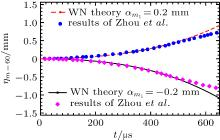Fig. 3.  Temporal evolution of mode m = 60 from initial two-mode perturbation (m1 = 40, m2 = 20). The solid and dashed lines are WN solutions to Eq. (16), the markers are the corresponding simulation results in Ref.  for αm1 = ± 0.2 mm and αm2 = 1.5 mm.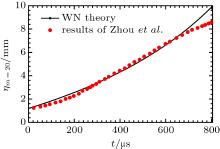Fig. 4.  Perturbation amplitude of mode m = 20 versus time for initial two-mode perturbation (m1 = 40, m2 = 20) with αm1 = –0.2 mm and αm2 = 1.5 mm. The solid line denotes our WN result, the dotted line is the numerical result taken from Ref. .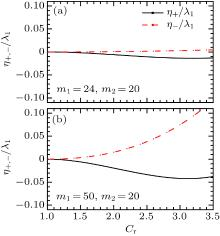Fig. 5.  The normalized amplitudes η+/λ1 (solid) and η–/λ1 (dashed) versus convergence ratio Cr for two nearby modes (m1 = 24, m1 = 20) (a) and dissimilar modes (m1 = 50, m1 = 20) (b) perturbations at the initial stage. The initial perturbations are ${\dot{\alpha }}_{{m}_{1}}={\dot{\alpha }}_{{m}_{2}}=0.01{V}_{0}$ and the Atwood number A = 1.0.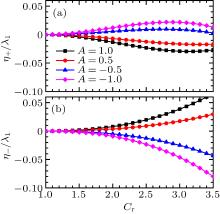Fig. 6.  The normalized amplitudes η+/λ1 (a) and η–/λ1 (b) for the Atwood numbers A = 1.0, 0.5, –0.5 and –1.0 with the initial perturbation ${\dot{\alpha }}_{{m}_{1}}={\dot{\alpha }}_{{m}_{2}}=0.01{V}_{0}$. The perturbation mode numbers are m1 = 40 and m2 = 20.